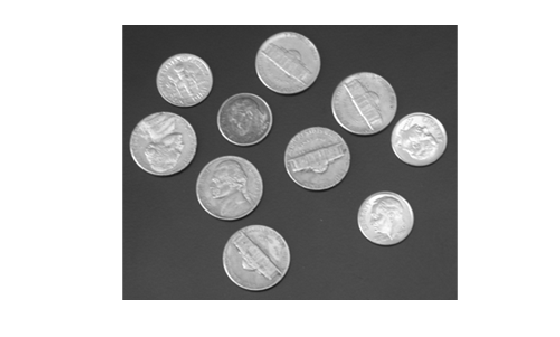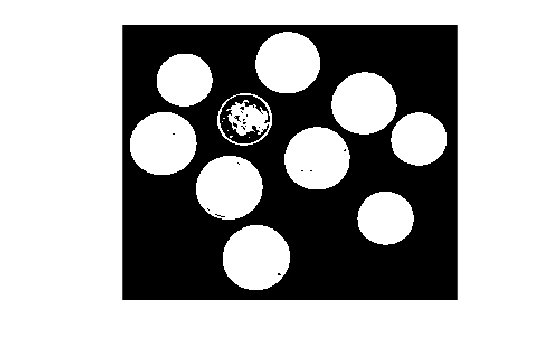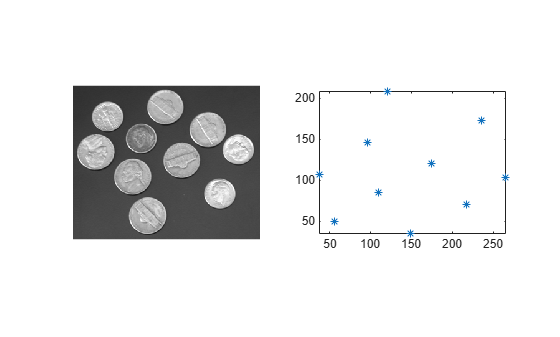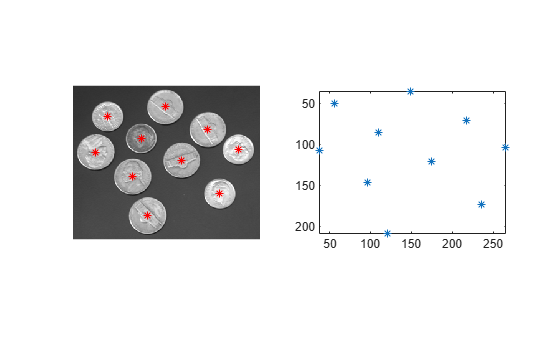# imgca

Get current axes containing image

## Syntax

``ax = imgca``
``ax = imgca(fig)``

## Description

example

````ax = imgca` returns the current axes that contains an image. The current axes can be in a regular figure window or in an Image Tool window. Note that the current axes that contains an image might not be the same as the most recently accessed axes.If no figure contains an axes that contains an image, then `imgca` creates a new axes.```
````ax = imgca(fig)` returns the current axes that contains an image in the specified figure.```

## Examples

collapse all

Read a grayscale image into the workspace.

```I = imread('coins.png'); imshow(I)```Convert the image into a binary image.

```bw = imbinarize(I); imshow(bw)```Fill holes in the binary objects, then calculate the centroids of the objects.

```bw2 = imfill(bw,'holes'); s = regionprops(bw2, 'centroid'); centroids = cat(1,s.Centroid);```

Display the original image and a plot of the centroids in the same figure window. Note that the current axes contains the plot of the centroids, not the displayed image.

```subplot(1,2,1) imshow(I) subplot(1,2,2) plot(centroids(:,1),centroids(:,2),'*') axis image```The direction of the y-axis is reversed for images. For equivalent comparison of the image and the plot of the centroids, reverse the y-axis direction of the plot. To get the most recent axes, which contains the plot of the centroids, use the `gca` function.

```h = gca; h.YDir = 'reverse';```

Use `imgca` to get the most recent axes containing an image. Note that this axes is not the most recent axes. Overlay the centroids in red asterisks on the image.

```hIm = imgca; hold(hIm,'on') plot(hIm,centroids(:,1),centroids(:,2),'r*') hold(hIm,'off')```## Input Arguments

collapse all

Figure, specified as a `figure` object.

## Output Arguments

collapse all

Current axes containing an image, returned as an `axes` object.

## Tips

• `imgca` can be useful in returning the axes object in the Image Tool. You cannot retrieve this axes using `gca`.